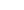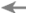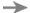# Leverage Point

## Definition

The concept of leverage is one of the most powerful in all of science. Archimedes proved the Law of the Lever and applied the law in a variety of inventions. Historian John Tzetzes, writing in the 12th century, wrote that Archimedes said "Give me a place to stand and with a lever I will move the world." 1In systems thinking a leverage point is a place in a system's structure where a solution element can be applied. It's a low leverage point if a small amount of change force causes a small change in system behavior. It's a high leverage point if a small amount of change force causes a large change in system behavior.

Change force is the effort required to prepare and make a change. In social problems the effort to prepare to make a change usually dwarfs the final effort to actually make the change. For example, in the civil rights problem it took decades of demonstrations, rallies, speeches, personal conversations, legislative lobbying, and new law proposals to prepare to make the change. To actually change the system required the mere signing of a new bill and then enforcing it.

## How do you find high leverage points?Finding the high leverage points for a difficult social problem requires the use of advanced problem solving tools. The ones we lean toward are shown.

In difficult problems, high leverage points resolve root causes. Why this is so is explained in the causal chain glossary entry.

Therefore the only reliable way to find high leverage points is to first find the root causes. Then, and only then, can you start looking for the high leverage points associated with each root cause.

So how do you find the root causes?

That's the hard part. It's the step that makes or breaks your entire problem solving effort. Get it right and the rest is relatively easy. Get it wrong, and no amount of hard work and cleverness will work, because activists simply do not have enough change force to push on low leverage points to get them to work.

If you haven't found the root causes, then your solutions are pushing on low leverage points in a vain effort to resolve intermediate causes. That's why they're not working.

Those working on the sustainability problem have been trying solution after solution for decades. While there have been some small successes, the overall verdict is these solutions have failed. That is clear strong evidence these are superficial solutions. They push on intermediate causes rather than root causes. That's why they are failing.

## The Analytical Activist's Problem Solving Map

Study the map below closely. It's a fragment from this poster. The map shows how to correctly follow the causal chain of a problem. When analyzing a difficult problem, follow the chain of cause and effect from problem symptoms all the way to root causes, high leverage points, and fundamental solutions. Don't make the mistake of taking a wrong turn at intermediate causes, like classic activists have.Here's why leverage is so important:

Symptomatic solutions attempt to push on the system at low leverage points to resolve intermediate causes. This works on easy problems because on easy problems the forces arising from root causes are small enough to be overcome by pushing on low leverage points.

Fundamental solutions push on the system at high leverage points to resolve root causes. This approach is required on difficult problems since problem solvers can exert only limited amounts of force on a large system. If that force is applied at low instead of high leverage points, it will be overwhelmed by the natural forces of the system that arise from the root causes.

## The Leverage Equation

In science leverage is the ratio of change in output to the change in input. The formula for calculating leverage is:

leverage = change in output / change in input

which is the same as:

leverage x change in input = change in output

This can be restated into a much more understandable form, one that advanced problem solvers need to memorize:

leverage x force = change

The amount of leverage times the amount of force
equals the amount of change to the system.

The equation says that if you want to succeed in solving a difficult problem, then you must study the system so that you can find the high leverage points that will turn your limited amount of change force into enough change to solve the problem. If the equation is true then no other way will work.

Note that pushing on the wrong leverage point will cause the wrong change. So the quality (correctness and efficiency) of the leverage point equates to the quality of the change. Garbage in, garbage out. Quality in, quality out.

## Leverage Efficiency (LE)

On February 17, 2019 Montserrat Koloffon and Jack Harich discovered the concept of leverage efficiency, as it applies to an SIP analysis. Leverage efficiency is a measure of how well pushing on a leverage point resolves a root cause. If it's a high leverage point, 100% LE is defined as enough force to resolve the root cause. A low leverage point will by definition be incapable of achieving 100% LE, and will generally achieve less than 50%.

Negative leverage efficiency occurs when pushing on a leverage point makes the problem worse instead of better. A famous example of negative LE may be found in Jay Forrester's book, Urban Dynamics, 1969. The system dynamics model in the book showed how, of the four leading solutions to solving the US urban decay problem, solution effectiveness ranged from zero impact to highly detrimental. The most popular solution of them all, low-cost housing, was the most detrimental and made the problem MUCH worse. In other words, it had high negative LE. For further reading, see How the Urban Decay Crisis Was Solved.

The concept of LE applies very well to how SIP approaches analysis. Low leverage points have low LE, and so are incapable of solving a problem. By contrast, high leverage points have high enough LE to resolve the root cause(s) and solve the problem. The terms solution leverage efficiency and leverage point efficiency now have precise and very useful meanings.

The effectiveness of various leverage points (such as low, medium, and high) can be easly compared by measuing their LEs. This allows quantitatively comparing the effectiveness of superficial and fundamental solutions (or past and proposed solutions) in a well-organized, easy to understand, credible manner.

## The Importance of Pushing on High Leverage PointsAs conceptually illustrated in the diagram, the choice of the correct high leverage point allows a small problem solving force to have a large effect on system behavior. This requires choosing the right lever and its application point. In social problems leverage is the use of indirect force, rather than direct force.

An example of a low leverage point would be pushing on the side of a ship to change its course. This would require a large amount of force to have the intended effect. But if the high leverage point of pushing on the rudder is used instead, it takes only a small amount of force to achieve the same effect.

Easy problems can be solved by pushing on low leverage points. Difficult problems require pushing on high leverage points, either because large amounts of change are required or to overcome large system change resistance.

Low leverage points attempt to solve intermediate causes. That can't work because it does nothing to resolve the cause of the intermediate causes, which is the root causes. In contrast, high leverage points resolve root causes. Seeing difficult social problems this way is the key to solving them.

At a favorable high leverage point a small structural change to a system can cause the system to behave much more favorably. Only the use of the correct high leverage points can solve a difficult complex social system problem, because if a low leverage point is used, system resistance cannot be overcome.

For example, environmentalists have long been pushing on the low leverage point of more of the truth. But they do not have enough force, in terms of numbers, influence, and wealth, to make pushing there work. Instead, they must find the high leverage points in the system and push there instead.

The concept of leverage points is so powerful that Peter Senge, in The Fifth Discipline: The Art and Practice of the Learning Organization, devotes an entire chapter to the subject. The chapter, titled The Principle of Leverage, opens with these words:

The bottom line of systems thinking is leverage—seeing where actions and changes in structures can lead to significant, enduring improvements. Often leverage follows the principle of economy of means: where the best results come not from large-scale efforts but from small well-focused actions. Our non-systematic ways of thinking are so damaging specifically because they consistently lead us to focus on low leverage changes: we focus on symptoms where the stress is greatest. We repair or ameliorate the symptoms. But such efforts only make matters better in the short run, at best, and worse in the long run.

It's hard to disagree with the principle of leverage. But the leverage in most real-life systems, such as most organizations, is not obvious to most of the actors in those systems. They don't see the 'structures' underlying their actions.

Peter closes the chapter with this observation:

The essence of mastering systems thinking as a management discipline lies in seeing patterns where others see only events and forces to react to.

## Avoiding the Trap of Intuitively Derived High Leverage Points

We will close with a different observation. When problem solvers are first exposed to the concept of high leverage points, a nearly universal phenomenon transpires: they start thinking and talking about "Where are the high leverage points? Is this one? No, probably not. Well, what about this one? Or this one?" Or they start calling their preferred solutions "high leverage solutions."

At that point what you are really doing is the same thing you were doing before: creating solution strategies intuitively. You are trying to brainstorm high leverage points, with is the same as brainstorming solutions.

If this happens to you, then it will help to realize that high leverage points are not identified by intuitive hard thinking. They are found by calm, prolonged true analysis, such as in the second step of the System Improvement Process. In that step, first you model the problem to find the root cause of the undesirable symptoms. Only after that do you start examining the model for high leverage points to resolve the root cause. If you've taken the time to build a good model, the high leverage points will be obvious. If you have not built a good model, or have skipped the analysis step, then the high leverage points will remain as elusive as ever.

(1) Archimedes quote from The Lever Quotations. Image of Archimedes moving the world from the Wikipedia entry on lever.

Browse the GlossaryPreviousNextRELATED INFORMATION
Leverage Points in the System Improvement Process

The only way to solve a difficult social problem is to push on high leverage points in order to resolve the problem's root causes. The System Improvement Process (SIP) employes leverage points this way:

First SIP decomposes the one big problem into three smaller subproblems. Then the analysis looks at each subproblem to find its root causes. Then SIP finds the low leverage points problem solvers have been pushing on in vain to solve the problem. Next SIP finds the feedback loops that should be dominant to resolve the root causes. Finally SIP finds the high leverage points needed to make those loops go dominant.

This may sound like a lot of work, but it's a lot less work that continuing to push on low leverage points in vain, which is what it appears sustainability problem solvers are doing now.